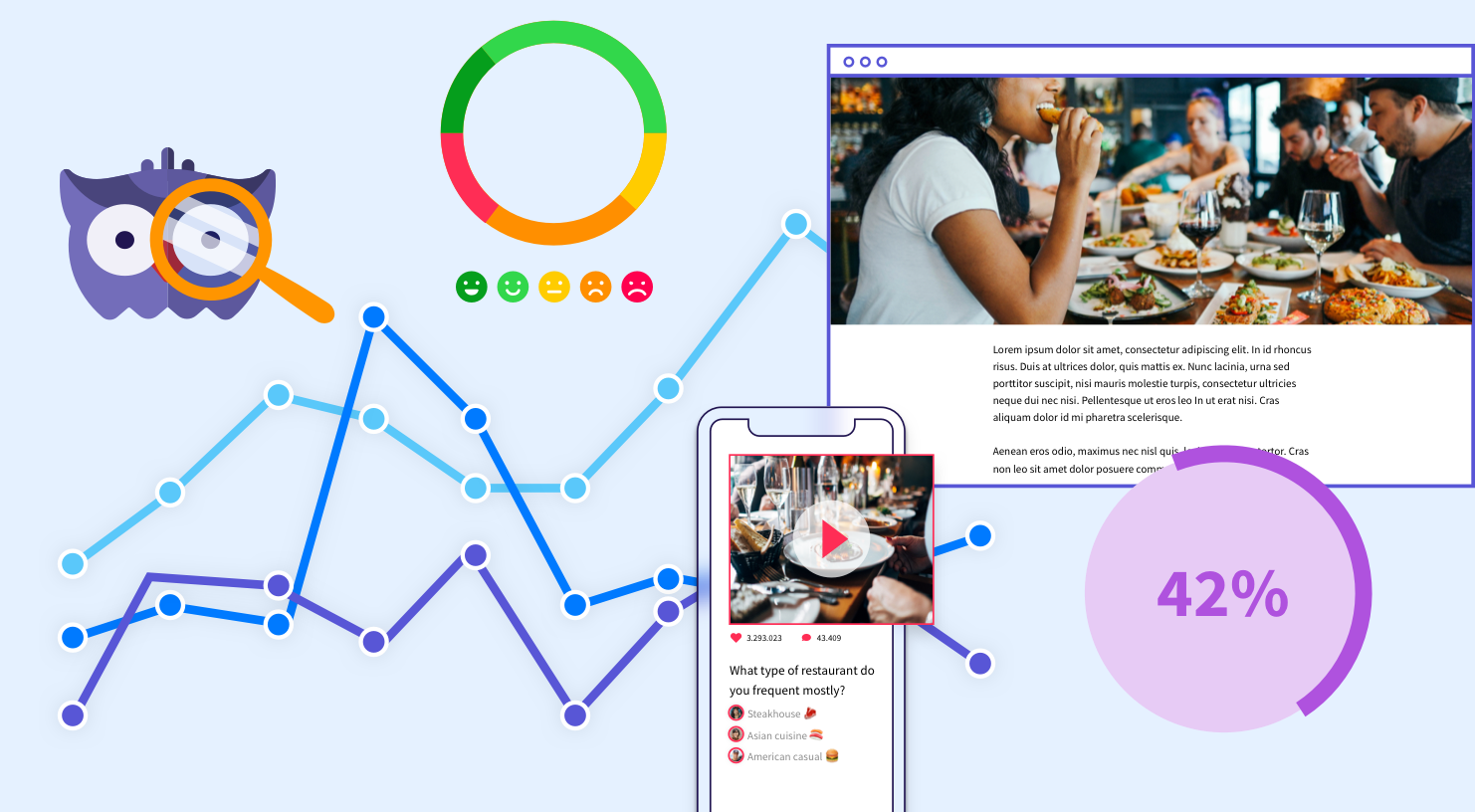# When Science Gets Involved in Politics

Naira Musallam, PhDJun 7 2016“You won’t give me the money to pay for a scientific poll” declared Roger Stone as he separated from the Donald Trump campaign as a senior advisor. What did he mean by that and why is it so important for the voter to know something about it?

There is no shortage of polling results presented on a daily basis. In fact, there are so many of them that many times there are even conflicting results.  Whether you are republican, democrat, or an independent voter, it is important to be able to weed out the good from the bad polling results so you can make informed decisions. How exactly do you do that?

Welcome to inferential statistics.  Sounds complicated and a bit out of your comfort zone?  It shouldn’t be, and here’s why. The principles are much simpler than you think.  In an ideal world, a public opinion poll would reach out to its entire population and ask them about their opinions.  This isn’t really feasible with over 200 million registered voters in the U.S. Because it is not feasible to do so, statisticians opt for sampling techniques; to select cases so the final sample is representative of the population from which it was drawn. A sample can be considered representative if it is able to replicate the important characteristics of the population.  For example, if a population consists of 60% female and 40% male, then a representative sample would have the same ratio composition. The sample should have the same proportional makeup of all important demographic characteristics such as age, location, socioeconomic status, and ethnic background. In other words, a representative sample is similar to the population but on a smaller scale.

How do we guarantee a representative sample? While we could never guarantee 100% representation, we are able to maximize the chances of a representative sample by following the principle of EPSEM (the “Equal Probability of Selection Method”), considered the fundamental principle of probability sampling. Statisticians have developed several sampling  EPSM techniques, including Simple Random sampling (cases are randomly drown from tables or lists), Systematic Random sampling (where a starting point is chosen at random and choices thereafter are at regular intervals), Stratified Random sampling (where you first divide the population list into sub-lists according to important characteristics and then sample from those lists), and Cluster sampling (which involves selecting groups of cases rather than single cases where the clusters are based on important characteristics such as geography).

The EPSM techniques are sound scientific techniques that increase the probability of having a representative sample. Once obtained, statisticians rely on estimation techniques, to estimate population voting based on sample statistics.

So how do we move from sample statistics to inference about the population? Another important concept is something we call Sampling Distribution. It is the distribution of a statistic (such as the mean) for all possible sample outcomes of a certain size.  What is important to understand here is that the sampling distribution is theoretical, meaning that the researcher never obtains it in reality, but it is critical for estimation. Why? This is due to its theoretical properties.  The first being its shape is normal.  You have heard before about the normal or “Bell Curve”, which is a theoretical distribution of scores that is symmetrical and bell shaped. The standard normal curve always has a mean of 0 and a standard deviation of 1.  Furthermore, there are known probabilities that can be calculated based on the mean and standard deviation.

Here are some interesting distributions of the normal curve:

• The distance between one standard deviation above the mean and one standard deviation below the mean encompasses exactly 68.26% of the total area under the curve
• The distance between two standard deviations above the mean and two standard deviations below the mean encompasses exactly 95.44% of the total area under the curve

Back to the sampling distribution. First, because one can assume its shape is normal, that leads to the ability to calculate probabilities of various outcomes, if the mean and standard deviation are known (after converting scores to standardized scores known as Z scores, which specify whether a specific score is below or above the mean and by how many standard deviations).

Second, the mean of the sampling distribution is the same value as the mean of the population, and the standard error is equal to the population standard deviation divided by the square root of N. This is a result of the Central Limit Theorem: if repeated random sample of size N is drawn from any population with mean and standard deviation, then as N becomes large, the sampling distribution of sample means will approach normality.

At the end of the day, U.S. voters need to pay attention to the sampling techniques used in these polls. If it isn’t one of the above, then there’s a good chance the results you’re looking at are a bit more misleading than you may think.  Once you know that the sampling technique is sound, it is worth paying attention to the sample size and the confidence intervals involved.## More from SightX### How to Deliver More Engaging Content With Insights

Content marketing is nothing new, but as many marketers already know- the rules just keep on changing.

bySavannah Trotter### How to Create More Effective Brand Awareness Surveys [Free Template]

Have you ever wondered how popular your brand is with consumers?

byLeila### What Are You Weighting For?

byNaira Musallam, PhD## Research Services